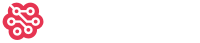## What percentage of my answers are accepted?

9

(click on this box to dismiss)

Returns the total number of answers, the number of accepted answers, and what percentage of answers are accepted.Q&A for users and developers of hardware and software for Raspberry Pi

``````-- What percentage of my answers are accepted?
-- Returns the total number of answers, the number of accepted answers, and what percentage of answers are accepted.

SELECT
Sum(CASE q.AcceptedAnswerId WHEN a.Id THEN 1 ELSE 0 END) AS [Accepted Answers],
Round(Sum(CASE q.AcceptedAnswerId WHEN a.Id THEN 1 ELSE 0 END) * 100.0 / Count(a.Id), 1) AS [Percentage Accepted]
FROM
Posts AS a
JOIN Posts AS q
ON a.ParentId = q.Id
WHERE
a.OwnerUserId = ##UserId##
AND q.postTypeId = 1
AND a.postTypeId = 2``````

### Enter Parameters

Options:
-Hold tight while we fetch your results
:records returned in :time ms:cached